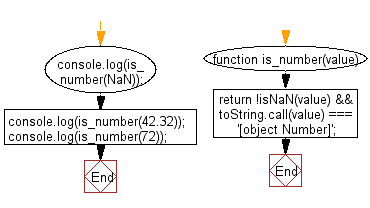# JavaScript: Validate whether a given value is number or not

## JavaScript validation: Exercise-5 with Solution

Write a JavaScript function to validate whether a given value is a number or not.

Sample Solution:-

HTML Code:

``````<!DOCTYPE html>
<html>
<meta charset="utf-8">
<title>JavaScript function to validate whether a given value is number or not.</title>
<body>

</body>
</html>
```
```

JavaScript Code:

``````function is_number(value)
{
return !isNaN(value) && toString.call(value) === '[object Number]';
}

console.log(is_number(NaN));

console.log(is_number(42.32));

console.log(is_number(72));
```
```

Sample Output:

```false
true
true
```

Flowchart:Live Demo:

See the Pen javascript-validation-exercise-5 by w3resource (@w3resource) on CodePen.

Improve this sample solution and post your code through Disqus

What is the difficulty level of this exercise?

Test your Programming skills with w3resource's quiz.

﻿